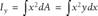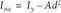# Calculating the Second Moment of an Area

Problem

You've learned how to compute area and center of area using numerical integration as discussed in the previous recipe and now you want to learn how to compute the second moment of the area.

Solution

Use the same technique as discussed in the previous recipe for computing the first moment of the area, but this time use the product of x2 and y instead of x and y.

Discussion

You can easily extend the example shown in Figure 10-2 from the previous recipe to include computing the second moment of the area. In mechanics, the second moment of an area is called the moment of inertia and is computed according to the following formula:This yields the moment of inertia about the y-axis. The formula for computing the second moment about the x-axis is very similar; the xs and ys are just swapped.

Often, you're more interested in the moment of inertia about an axis that passes through the center of an area. In that case you need to apply the well-known parallel axis theorem:Here, Ina is the moment of inertia of the area about an axis parallel to the y-axis, but passing through the centroid of the area. A is the area, and d is the distance from the y-axis to the axis passing through the centroid.

Figure 10-2 includes the results of these computations for the area considered earlier. Cell C23 computes the moment of inertia about the y-axis using the formula =C17/3*SUMPRODUCT(C5:C15,B5:B15^2,D5:D15). This formula looks very similar to that shown earlier for computing the first moment of the area. However, in this case we square the x-values using the argument B5:B15^2.

Application of the parallel axis theorem yields the moment of inertia about an axis that passes through the center of the area. Cell C24 computes this value using the formula =C23-C19*C20^2. In this case, the d term from the parallel axis theorem is the distance along the x-axis to the center of area, which was already computed in the example from the previous recipe.

Read Recipe 10.3 for more information on applying Simpson's rule to calculate areas and centers of areas.Excel Scientific and Engineering Cookbook (Cookbooks (OReilly))
ISBN: 0596008791
EAN: 2147483647
Year: N/A
Pages: 206
Authors: David M Bourg